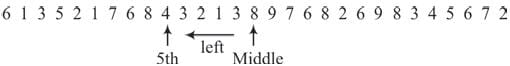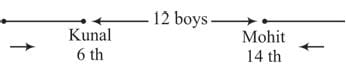# Olympiad Test:Number Ranking - 2

## 10 Questions MCQ Test Science Olympiad Class 8 | Olympiad Test:Number Ranking - 2

Description
Attempt Olympiad Test:Number Ranking - 2 | 10 questions in 20 minutes | Mock test for Class 8 preparation | Free important questions MCQ to study Science Olympiad Class 8 for Class 8 Exam | Download free PDF with solutions
QUESTION: 1

### Rohit is 14th from the right end in a row of 70 students. What is his position from left end?

Solution:

Numbers of students towards left to Rohit = Rohit’s position from left = 56 + 1 = 57th

QUESTION: 2

### Manak ranks 5th from the top and third seventh from the bottom in a class, How many students are there in the class?

Solution:

Total number of students = 4 + 1 + 36 = 41.

QUESTION: 3

### Which is the 5th number to the left of the number which is exactly in the middle of the given sequence of numbers? 6 1 3 5 21 7 6 8 4 3 2 1 3 8 9 7 6 8 2 6 9 8 3 45 6 7 2

Solution:Total numbers = 29.
Middle number = 15th number

QUESTION: 4

How many numbers from 50 to 150 are there each of which is exactly divisible by 4 and also has 4 as a digit?

Solution:

The numbers divisible by 4 from 50 to 150 are 52, 56, 60, 64, 68, 72, 76, 80, 84, 88, 92, 96, 100,104, 108, 112, 116, 120, 124, 128, 132, 136, 140, 144, 148.
The numbers which has 4 as a digit are 64, 84, 104, 124, 140, l44, 148

QUESTION: 5

How many numbers from 1 to 100 are there which are exactly divisible by 7 but not by 3?

Solution:

The numbers from 1 to 100 which are divisible by 7 but not by 3 are
7, 14, 28, 35, 49, 56, 70, 77, 91, 98,

QUESTION: 6

Ranjit ranked fifteenth from the top and fourty-sixth from the bottom in a class. How many students are there in the class?

Solution:

Total number of students = 14 + 1 + 45 = 60

QUESTION: 7

Sunita ranks fifteenth in a class of 75 students. What is her rank from the last?

Solution:

Number of students behind Sunita in rank = 75 – 15 = 60.
Sunita’s rank from last = 60 + 1 = 61

QUESTION: 8

In a class of 45 students, Raju is 7th from the bottom, where as Samir is placed 9th from the top. Peter is placed exactly in between two. What is Raju’s position from Peter?

Solution:

Number of students between Raju and Samir = 45 – (7 + 9) = 45 – 16 = 29

Peter is 15th in between Raju and Samir. Raju’s position is 15th from Peter.

QUESTION: 9

Kunal is 6th from the left end and Mohit is 14th from the right end in a row of boys. If there are 12 boys between Kunal and Mohit then how many boys are there in the row?

Solution:Total number of boys = 6 + 12 + 14 = 32

QUESTION: 10

In a row of boys if Shankar is 10th from the left and Mahesh is 9th from the right interchange their positions, Shankar becomes15th from the left. How many students are there in the row?

Solution:

Number of students in the row = (15 + 9) – 1 = 24 – 1 = 23Use Code STAYHOME200 and get INR 200 additional OFF Use Coupon Code